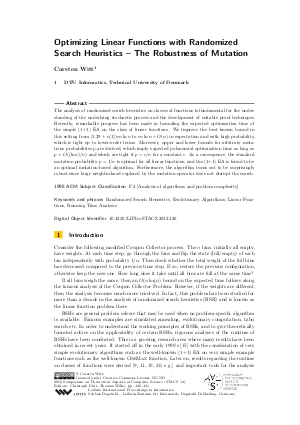Document# Optimizing Linear Functions with Randomized Search Heuristics - The Robustness of Mutation

### Author Carsten Witt## File

LIPIcs.STACS.2012.420.pdf
• Filesize: 0.61 MB
• 12 pages

## Cite As

Carsten Witt. Optimizing Linear Functions with Randomized Search Heuristics - The Robustness of Mutation. In 29th International Symposium on Theoretical Aspects of Computer Science (STACS 2012). Leibniz International Proceedings in Informatics (LIPIcs), Volume 14, pp. 420-431, Schloss Dagstuhl - Leibniz-Zentrum für Informatik (2012)
https://doi.org/10.4230/LIPIcs.STACS.2012.420

## Abstract

The analysis of randomized search heuristics on classes of functions is fundamental for the understanding of the underlying stochastic process and the development of suitable proof techniques. Recently, remarkable progress has been made in bounding the expected optimization time of the simple (1+1) EA on the class of linear functions. We improve the best known bound in this setting from (1.39+o(1))(en ln n) to (en ln n)+O(n) in expectation and with high probability, which is tight up to lower-order terms. Moreover, upper and lower bounds for arbitrary mutations probabilities p are derived, which imply expected polynomial optimization time as long as p=O((ln n)/n) and which are tight if p=c/n for a constant c. As a consequence, the standard mutation probability p=1/n is optimal for all linear functions, and the (1+1) EA is found to be an optimal mutation-based algorithm. Furthermore, the algorithm turns out to be surprisingly robust since large neighborhood explored by the mutation operator does not disrupt the search.
##### Keywords
• Randomized Search Heuristics
• Evolutionary Algorithms
• Linear Functions
• Running Time Analysis

## Metrics

• Access Statistics
• Total Accesses (updated on a weekly basis)
0